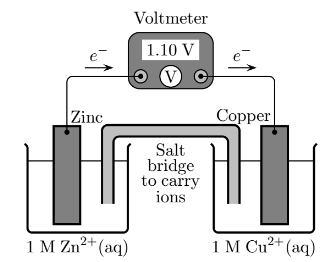# Problem: In this electrochemical cell, what is the anode? 1. The solid zinc electrode  2. The Zn2+(aq) ions in the 1 M solution 3. The Cu2+(aq) ions in the 1 M solution 4. The solid copper electrode

###### FREE Expert Solution
89% (405 ratings)
###### Problem Details

In this electrochemical cell, what is the anode?

1. The solid zinc electrode

2. The Zn2+(aq) ions in the 1 M solution

3. The Cu2+(aq) ions in the 1 M solution

4. The solid copper electrodeWhat scientific concept do you need to know in order to solve this problem?

Our tutors have indicated that to solve this problem you will need to apply the Galvanic Cell concept. You can view video lessons to learn Galvanic Cell. Or if you need more Galvanic Cell practice, you can also practice Galvanic Cell practice problems.

What is the difficulty of this problem?

Our tutors rated the difficulty ofIn this electrochemical cell, what is the anode? 1. The sol...as medium difficulty.

How long does this problem take to solve?

Our expert Chemistry tutor, Sabrina took 2 minutes and 7 seconds to solve this problem. You can follow their steps in the video explanation above.

What professor is this problem relevant for?

Based on our data, we think this problem is relevant for Professor Lavelle's class at UCLA.